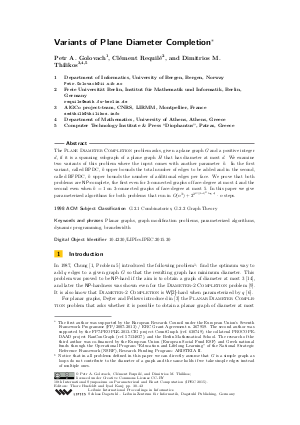Document# Variants of Plane Diameter Completion

### Authors Petr A. Golovach, Clément Requilé, Dimitrios M. Thilikos## File

LIPIcs.IPEC.2015.30.pdf
• Filesize: 0.62 MB
• 13 pages

## Cite As

Petr A. Golovach, Clément Requilé, and Dimitrios M. Thilikos. Variants of Plane Diameter Completion. In 10th International Symposium on Parameterized and Exact Computation (IPEC 2015). Leibniz International Proceedings in Informatics (LIPIcs), Volume 43, pp. 30-42, Schloss Dagstuhl - Leibniz-Zentrum für Informatik (2015)
https://doi.org/10.4230/LIPIcs.IPEC.2015.30

## Abstract

The Plane Diameter Completion problem asks, given a plane graph G and a positive integer d, if it is a spanning subgraph of a plane graph H that has diameter at most d. We examine two variants of this problem where the input comes with another parameter k. In the first variant, called BPDC, k upper bounds the total number of edges to be added and in the second, called BFPDC, k upper bounds the number of additional edges per face. We prove that both problems are NP-complete, the first even for 3-connected graphs of face-degree at most 4 and the second even when k=1 on 3-connected graphs of face-degree at most 5. In this paper we give parameterized algorithms for both problems that run in O(n^{3})+2^{2^{O((kd)^2\log d)}} * n steps.
##### Keywords
• Planar graphs
• graph modification problems
• parameterized algorithms
• dynamic programming
• branchwidth

## Metrics

• Access Statistics
• Total Accesses (updated on a weekly basis)
0